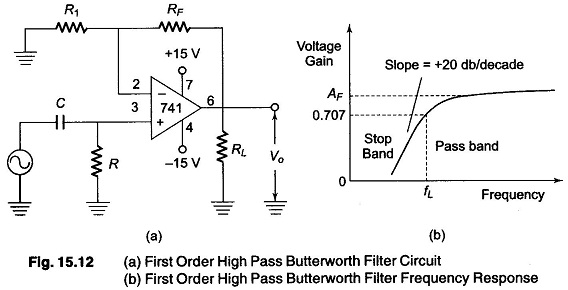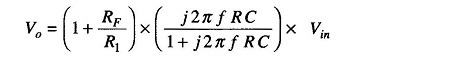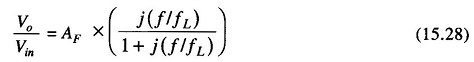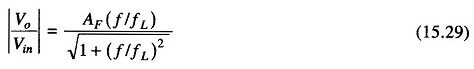## First Order High Pass Butterworth Filter Derivation:

First Order High Pass Butterworth Filter Derivation – High pass filters are often formed by interchanging frequency determining resistors and capacitors in a low pass filter.

A first order high pass filter is formed from a first order low pass type by interchanging components R and C.

Figure 15.12 shows a first order high pass Butterworth filter with a low cutoff frequency fL, the frequency at which the magnitude of the gain is 0.707 times its pass band value. All frequencies higher than fL are in the pass band with the highest frequency determined by the closed loop band width of the opamp.For the first order high pass filter, the output voltage isorwhere

• AF = 1 + RF/R– pass band gain of the filter
• f = frequency of the input signal
• fL= 1/(2 π R C) – low cutoff frequency

Hence the magnitude of the voltage gain isThe frequency scaling procedures are the same as for the low pass filter because high pass filters are formed from low pass filters by simply inter­changing Rs and Cs.

Scroll to Top